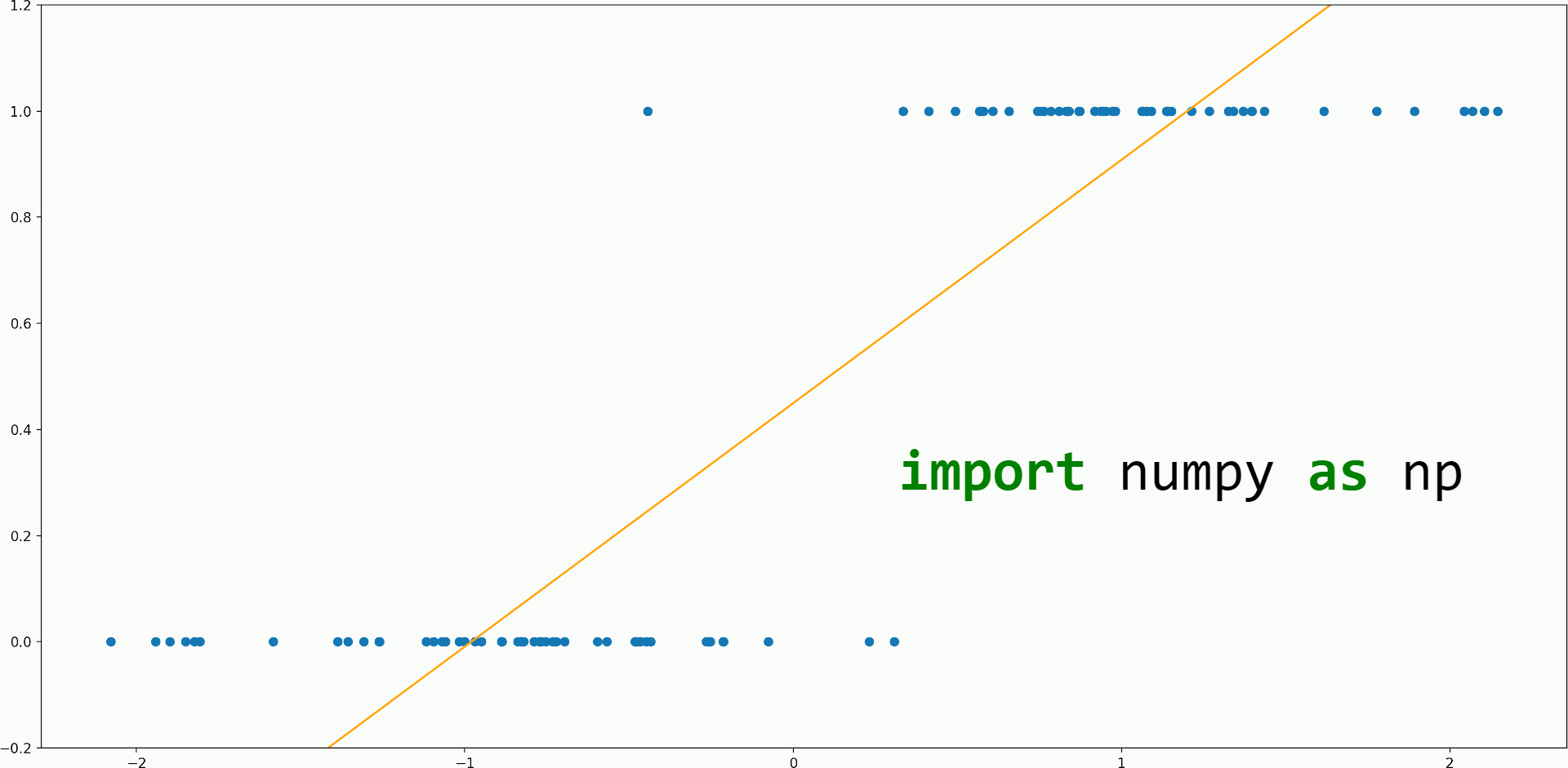# How to code Logistic Regression from scratch with NumPyHow to code Logistic Regression from scratch with NumPy. Sharpen your NumPy skills while learning Logistic Regression

What’s our plan for implementing Logistic Regression in NumPy?

Let’s first think of the underlying math that we want to use.

There are many ways to define a loss function and then find the optimal parameters for it, among them, here we will implement in our `LogisticRegression` class the following 3 ways for learning the parameters:

• We will rewrite the logistic regression equation so that we turn it into a least-squares linear regression problem with different labels and then, we use the closed-form formula to find the weights:• Like above, we turn logistic into least-squares linear regression, but instead of the closed-form formula, we use stochastic gradient descent with the following gradient:• We use the maximum likelihood estimation (MLE) method, write the likelihood function, play around with it, restate it as a minimization problem, and apply SGD with the following gradient:In the above equations, X is the input matrix that contains observations on the row axis and features on the column axis; y is a column vector that contains the classification labels (0 or 1); f is the sum of squared errors loss function; h is the loss function for the MLE method.

## Data Science With Python Training | Python Data Science Course | Intellipaat

🔵 Intellipaat Data Science with Python course: https://intellipaat.com/python-for-data-science-training/In this Data Science With Python Training video, you...

## Data Science Projects | Data Science | Machine Learning | Python

Practice your skills in Data Science with Python, by learning and then trying all these hands-on, interactive projects, that I have posted for you.

## Data Science Projects | Data Science | Machine Learning | Python

Practice your skills in Data Science with Python, by learning and then trying all these hands-on, interactive projects, that I have posted for you.

## Data Science Projects | Data Science | Machine Learning | Python

Practice your skills in Data Science with Python, by learning and then trying all these hands-on, interactive projects, that I have posted for you.

## Data Science Projects | Data Science | Machine Learning | Python

Practice your skills in Data Science with Python, by learning and then trying all these hands-on, interactive projects, that I have posted for you.